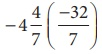Home | | Maths 8th Std | Rational numbers on a number line

# Rational numbers on a number line

Locating the rational numbers on a number line is an important skill.

Rational numbers on a number line

Locating the rational numbers on a number line is an important skill. For example, to represent the number 3/4 on the number line, 3/4 being negative would be marked to the left of 0 and it is between 0 and –1. We know that the integers, 1 and –1 are equidistant from 0 and so are the numbers 2 and –2, 3 and –3 from 0. This concept remains the same or rational numbers too. Now, as we mark 3/4 to the right of zero, at 3 parts out of 4 between 0 and 1, the same way, we will mark −3/4 to the left of zero, at 3 parts out of 4 between 0 and –1 as shown below.Similarly, it is easy to find -3/2 between –1 and –2 since -3/2 = - 1 ½ P

Now, on the following number line what rational numbers do the letters A and B represent?You will now be able to say easily the rational numbers marked by A and B on the number line as shown above. Isn’t it? Here, A represents the rational numberand B represents the rational numberTags : Numbers | Chapter 1 | 8th Maths , 8th Maths : Chapter 1 : Numbers
Study Material, Lecturing Notes, Assignment, Reference, Wiki description explanation, brief detail
8th Maths : Chapter 1 : Numbers : Rational numbers on a number line | Numbers | Chapter 1 | 8th Maths